#### Chapter 23 Graphical Representation of Statistical Data R.D. Sharma Solutions for Class 9th Exercise 23.3

Exercise 23.3

1. Construct a histogram for the following data: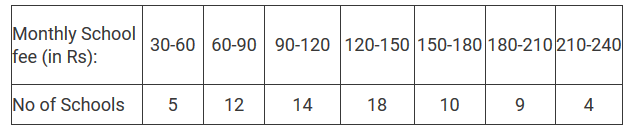Solution

To represent the given data by a histogram, we first draw horizontal and vertical axes. Let us consider that the horizontal and vertical axes represent the class-limits and the frequencies of the class-intervals respectively.
The given data is a continuous grouped frequency distribution with equal class-intervals. Construct rectangles with class-intervals as bases and respective frequencies as heights. It should be noted that the scale for horizontal axis may not be same as the scale for vertical axis. Let us take one vertical division is equal to 3 rupees.

The heights of the different rectangles are as following .

1. The height of the rectangle corresponding to the class-interval 30-60 is 5/3 = 1.66 big divisions.
2. The height of the rectangle corresponding to the class-interval 60-90 is 12/3 = 4 big divisions.
3. The height of the rectangle corresponding to the class-interval 90-120 is 14/3 = 4.66 big divisions.
4. The height of the rectangle corresponding to the class-interval 120-150 is 18/3 = 6 big divisions.
5. The height of the rectangle corresponding to the class-interval 150-180 is 10/3 = 3.33 big divisions.
6. The height of the rectangle corresponding to the class-interval 180-210 is 9/3 = 3 big divisions.
7. The height of the rectangle corresponding to the class-interval 210-240 is 4/3 = 1.33 big divisions.

The histogram of the given data is the following:-2. The distribution of heights (in cm) of 96 children is given below. Construct a histogram and a frequency polygon on the same axes.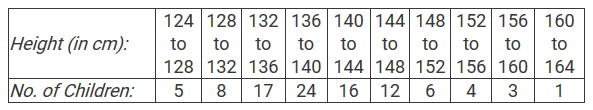Solution

To represent the given data by a histogram, we first draw horizontal and vertical axes. Let us consider that the horizontal and vertical axes represent the class-limits and the frequencies of the class-intervals respectively.

The given data is a continuous grouped frequency distribution with equal class-intervals. Construct rectangles with class-intervals as bases and respective frequencies as heights.
To draw the frequency polygon of the given data using histogram, obtain the mid-points of the upper horizontal side of each rectangle and then join these mid-points of the adjacent rectangles of the histogram by line segments. Obtain the mid-points of two class-intervals of 0 frequencies, i.e. on the horizontal axis, one adjacent to the first, on its left and one adjacent to the last, on its right. These class-intervals are known as imagined class-intervals. Complete the polygon by joining the mid-points of first and last class-intervals to the mid-points of imagined class-intervals adjacent to them. Let us take one vertical division is equal to 4.

The heights of the different rectangles are as following:-

1. The height of the rectangle corresponding to the class-interval 124-128 is 5/4 = 1.25 big divisions.
2. The height of the rectangle corresponding to the class-interval 128-132 is 8/4 = 2 big divisions .
3. The height of the rectangle corresponding to the class-interval 132-136 is 17/4 = 4.25 big divisions.
4. The height of the rectangle corresponding to the class-interval 136-140 is 24/4 = 6 big divisions .
5. The height of the rectangle corresponding to the class-interval 140-144 is 16/4 = 4 big divisions.
6. The height of the rectangle corresponding to the class-interval 144-148 is 12/4 = 3 big divisions .
7. The height of the rectangle corresponding to the class-interval 148-152 is 6/4 = 1.5 big divisions .
8. The height of the rectangle corresponding to the class-interval 152-156 is 4/4 = 1 big divisions .
9. The height of the rectangle corresponding to the class-interval 156-160 is 3/4 = 0.75 big divisions .
10.The height of the rectangle corresponding to the class-interval 160-164 is 1/4 = 0.25 big divisions

The histogram and frequency polygon of the given data is the following:3. The time taken, in seconds, to solve a problem by each of 25 pupils is as follows:
16, 20, 26, 27, 28, 30, 33, 37, 38, 40, 42, 43, 46, 46, 46, 48, 49, 50, 53, 58, 59, 60, 64, 52, 20
(a) Construct a frequency distribution for these data, using a class interval of 10 seconds.
(b) Draw a histogram to represent the frequency distribution.

Solution

Given that the times (in seconds) taken to solve a problem by each of 25 pupils are 16, 20, 26, 27, 28, 30, 33, 37, 38, 40, 42, 43, 46, 46, 46, 48, 49, 50, 53, 58, 59, 60, 64, 52 and 20. The minimum and maximum time values are 16 and 64 respectively.

(a) At first construct the following frequency distribution for the given data. Since, the lowest value is 16; we start with the class-interval 15-25, as the class size must be 10.(b) To represent the given data by a histogram, we first draw horizontal and vertical axes. Let us consider that the horizontal and vertical axes represent the class-limits and the frequencies of the class-intervals respectively.
The above data is a continuous grouped frequency distribution with equal class-intervals, which is 10. Construct rectangles with class-intervals as bases and respective frequencies as heights.

The histogram of the data in part (a) is as follows:4. Draw, in the same diagram, a histogram and a frequency polygon to represent the following data which shows the monthly cost of living index of a city in a period of 2 years: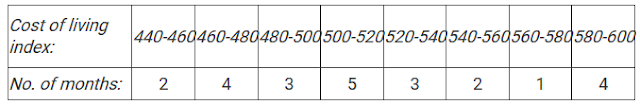Solution

To represent the given data by a histogram, we first draw horizontal and vertical axes. Let us consider that the horizontal and vertical axes represent the class-limits and the frequencies of the class-intervals respectively.

The given data is a continuous grouped frequency distribution with equal class-intervals. Construct rectangles with class-intervals as bases and respective frequencies as heights. It should be noted that the scale for horizontal axis may not be same as the scale for vertical axis. To draw the frequency polygon of the given data using histogram, obtain the mid-points of the upper horizontal side of each rectangle and then join these mid-points of the adjacent rectangles of the histogram by line segments. Obtain the mid-points of two class-intervals of 0 frequencies, i.e. on the horizontal axis, one adjacent to the first, on its left and one adjacent to the last, on its right. These class-intervals are known as imagined class-intervals. Complete the polygon by joining the mid-points of first and last class-intervals to the mid-points of imagined class-intervals adjacent to them. Let us take one vertical division is equal to 1 month.

The heights of the different rectangles are as follows:

1. The height of the rectangle corresponding to the class-interval 440-460 is 2 big divisions.
2. The height of the rectangle corresponding to the class-interval 460-480 is 4 big divisions.
3. The height of the rectangle corresponding to the class-interval 480-500 is 3 big divisions.
4. The height of the rectangle corresponding to the class-interval 500-520 is 5 big divisions.
5. The height of the rectangle corresponding to the class-interval 520-540 is 3 big divisions.
6. The height of the rectangle corresponding to the class-interval 540-560 is 2 big divisions.
7. The height of the rectangle corresponding to the class-interval 560-580is 1 big division.
8. The height of the rectangle corresponding to the class-interval 580-600 is 4 big divisions.

The histogram and frequency polygon of the given data is as follows:-5. The following is the distribution of total household expenditure (in Rs.) of manual worker in a city:Draw a histogram and a frequency polygon representing the above data.

Solution

To represent the given data by a histogram, we first draw horizontal and vertical axes. Let us consider that the horizontal and vertical axes represent the class-limits and the frequencies of the class-intervals respectively.
The given data is a continuous grouped frequency distribution with equal class-intervals. Construct rectangles with class-intervals as bases and respective frequencies as heights. It should be noted that the scale for horizontal axis may not be same as the scale for vertical axis. To draw the frequency polygon of the given data using histogram, obtain the mid-points of the upper horizontal side of each rectangle and then join these mid-points of the adjacent rectangles of the histogram by line segments. Obtain the mid-points of two class-intervals of 0 frequencies, i.e. on the horizontal axis, one adjacent to the first, on its left and one adjacent to the last, on its right. These class-intervals are known as imagined class-intervals. Complete the polygon by joining the mid-points of first and last class-intervals to the mid-points of imagined class-intervals adjacent to them. Let us take one vertical division is equal to 5 workers.

The heights of the different rectangles are as follows:-

1. The height of the rectangle corresponding to the class-interval 100-150 is 25/5 = 5 big divisions.
2. The height of the rectangle corresponding to the class-interval 150-200 is 40/5 = 8 big divisions.
3. The height of the rectangle corresponding to the class-interval 200-250 is 33/5 = 6.6 big divisions.
4. The height of the rectangle corresponding to the class-interval 250-300 is 28/5 = 28/5 = 5.6 big divisions.
5. The height of the rectangle corresponding to the class-interval 300-350 is 30/5 = 30/5 = 6 big divisions.
6 The height of the rectangle corresponding to the class-interval 350-400  is 22/5 = 4.4 big divisions.
7. The height of the rectangle corresponding to the class-interval 400-450 is 16/5 = 3.2 big divisions.
8. The height of the rectangle corresponding to the class-interval 450-500 is 8/5 = 1.6 big divisions.

The histogram of the given data is as follows:6. The following table gives the distribution of IQ's (intelligence quotients) of 60 pupils of class V in a school: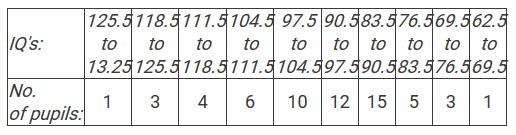Draw a frequency polygon for the above data.

Solution

We first draw horizontal and vertical axes. Let us consider that the horizontal and vertical axes represent the class-limits and the frequencies of the class-intervals respectively.
The given data is a continuous grouped frequency distribution with equal class-intervals. To draw the frequency polygon of the given data without using histogram, obtain the class-limits of the class intervals. Obtain the class-limits of two class-intervals of 0 frequencies, i.e. on the horizontal axis, one adjacent to the first, on its left and one adjacent to the last, on its right. These class-intervals are known as imagined class-intervals. Then plot the frequencies against class-limits.

The following table is useful to draw the frequency polygon of the given data.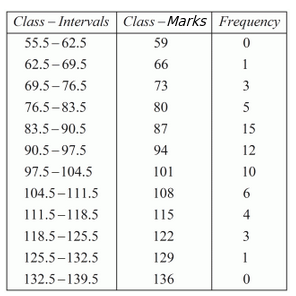We represent class marks on X-axis on a suitable scale and the frequencies on Y-axis on a suitable scale.

To obtain the frequency polygon we plot the points (66, 1), (73, 3), (80, 5), (87, 15), (94, 12), (101, 10), (108, 6), (115, 4), (122, 3), (129, 1).
Now we join the plotted points by line segments. The end points (66, 1) and (129, 1) are joined to the mid points (59, 0) and ( 136, 0) respectively of imagined class intervals to obtain the frequency polygon.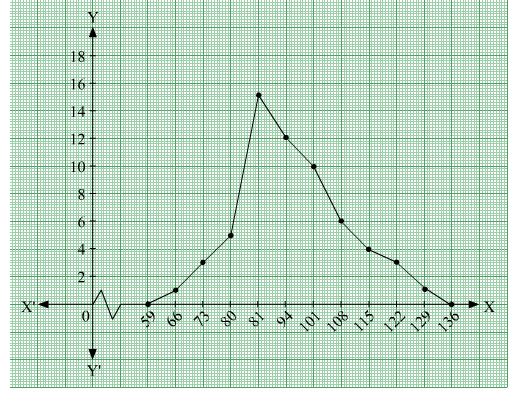7. Draw a histogram for the daily earnings of 30 drug stores in the following table:Solution

To represent the given data by a histogram, we first draw horizontal and vertical axes. Let us consider that the horizontal and vertical axes represent the class-intervals and the frequencies of the class-intervals respectively.
The given data is a continuous grouped frequency distribution with equal class-intervals. Construct rectangles with class-intervals as bases and respective frequencies as heights. The scale for horizontal axis may not be same as the scale for vertical axis. Let us take one vertical division is equal to 1 unit.

The heights of the different rectangles are as follows:

1. The height of the rectangle corresponding to the class-interval 450-500 is 16 big divisions.
2. The height of the rectangle corresponding to the class-interval 500-550 is 10 big divisions.
3. The height of the rectangle corresponding to the class-interval 550-600 is 7 big divisions.
4. The height of the rectangle corresponding to the class-interval 600-650 is 3 big divisions.
5. The height of the rectangle corresponding to the class-interval 650-700is 1 big divisions.

The histogram of the given data is as follows: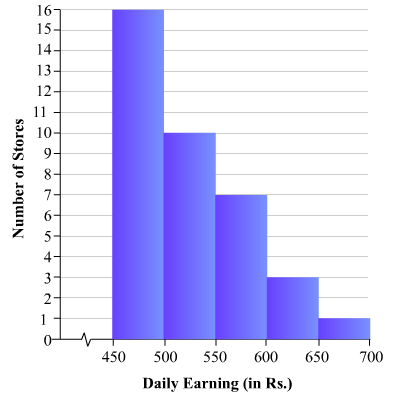8. The monthly profits (in Rs.) of 100 shops are distributed as follows:Draw a histogram for the data and show the frequency polygon for it.

Solution

To represent the given data by a histogram, we first draw horizontal and vertical axes. Let us consider that the horizontal and vertical axes represent the class-intervals and the frequencies of the class-intervals respectively.
The given data is a continuous grouped frequency distribution with equal class-intervals. Construct rectangles with class-intervals as bases and respective frequencies as heights. The scale for horizontal axis may not be same as the scale for vertical axis. Let us take one vertical division is equal to 3 shops.

The heights of the different rectangles are as following

1.The height of the rectangle corresponding to the class-interval 0-50 is 12/3 = 4 big divisions.
2.The height of the rectangle corresponding to the class-interval 50-100 is 18/3 = 6 big divisions.
3.The height of the rectangle corresponding to the class-interval 100-150 is 27/3 = 9 big divisions.
4.The height of the rectangle corresponding to the class-interval 150-200 is 20/3 = 6.67 big divisions.
5.The height of the rectangle corresponding to the class-interval 200-250 is 17/3 = 5.67 big divisions.
6.The height of the rectangle corresponding to the class-interval 250-300 is 6/3 = 2 big divisions .

The histogram of the given data is as follows:For frequency polygon, first we will obtain the class marks as given in the following table.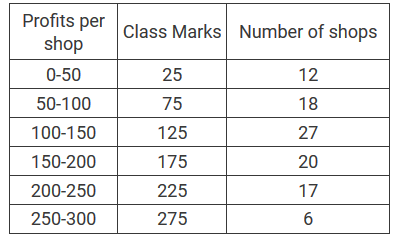We plot the points (25, 12), (75, 18), (125, 27), (175, 20), (225, 17) and (275, 6).
Now, we join the plotted points by line segments . The end points (25, 12) and (275, 6) are joined to the mid-points (−25, 0) and (325, 0) respectively of imagined class-intervals to obtain the frequency polygon.

The frequency polygon of the given data is as follows: